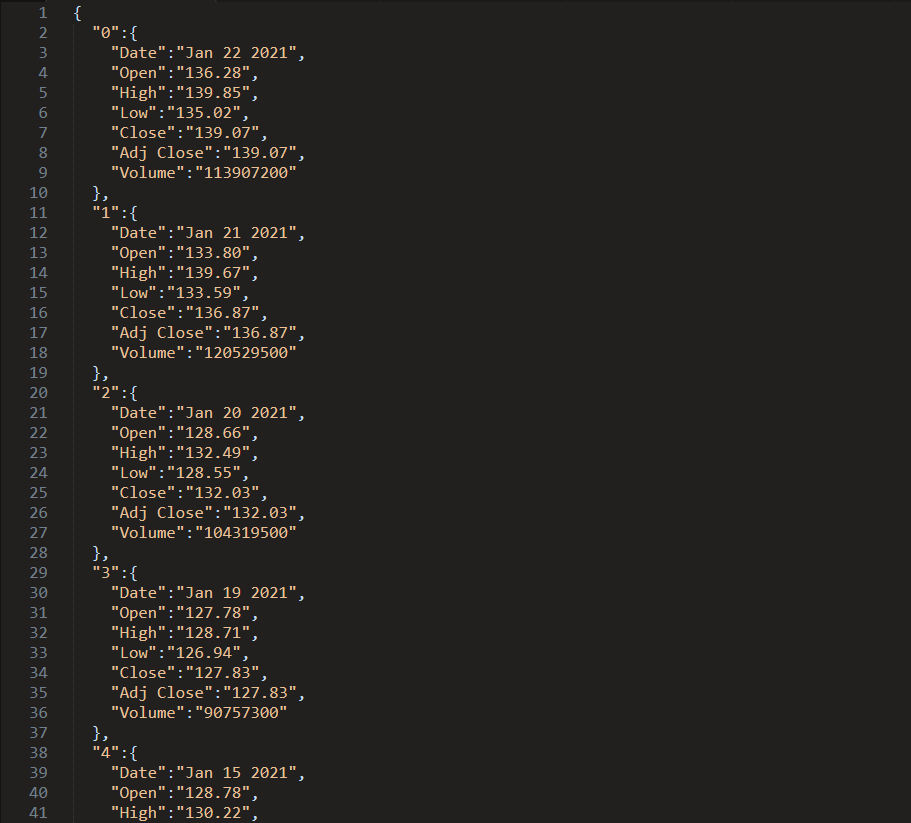signed

QiShunwang

“诚信为本、客户至上”

# 使用Python的pandas模块、mplfinance模块、matplotlib模块绘制K线图

2021/1/28 16:51:50   来源：

• pandas模块、mplfinance模块和matplotlib模块介绍
• pandas模块
• mplfinance模块和matplotlib模块
• 安装mplfinance模块、pandas模块和matplotlib模块
• 处理股票数据
• 设置图像大小
• 加载K线图并设置格式
• 完整代码

## pandas模块、mplfinance模块和matplotlib模块介绍

### pandas模块

pandas为解决数据分析任务而创建，纳入了大量的库和一些标准的数据模型，简而言之，它提供了很多数据处理的方法，此文就是借用它来生成DataFrame格式的数据。

### mplfinance模块和matplotlib模块

mplfinance是专用于金融数据的可视化分析模块，是基于matplotlib的实用模块程序，而matplotlib模块是python绘图领域使用最广泛的套件。简而言之，python想使用可视化，就可以使用matplotlib，想在可视化里面绘制金融方面的图标，就可以使用mplfinance。

## 处理股票数据

``````# k线图数据处理
# 读取json文件,获取数据

# 删除无效数据数据
for index, row in df.iterrows():
if(isnot_float(row['Open'])):
df.drop(index, inplace=True)

# 调整数据顺序
df = df.iloc[::-1]

# 设置索引
df.set_index(['Date'], inplace=True)
# 将数据列转换为float,因为json字符串存在坏数据，所以读取的时候，所有列默认为字符串了。
df = df.apply(pd.to_numeric, errors='ignore')
``````## 设置图像大小

``````# 绘图
# 自定义一个10*8的画布
fig = plt.figure(figsize=(12, 8))
# 对画布划分，显示在1行*1列的第一块区域，其实也仅有一块区域
``````

## 加载K线图并设置格式

``````# 加载K线图
mpf.plot(df, type='candle', ax=ax, style='binance', datetime_format='%Y-%m-%d')
# 设置横轴值为时间格式
ax.xaxis_date()
# 通过tight_layout(),实现子图集铺满
plt.tight_layout()
# 显示
plt.show()
``````

## 完整代码

``````import matplotlib.pyplot as plt
import mplfinance as mpf
import pandas as pd

# 用来判断str能否转换为float，用于剔除无效数据
def isnot_float(str):
try:
float(str)
return False
except ValueError:
print(str)
return True

# k线图数据处理
# 读取json文件,获取数据

# 删除无效数据数据
for index, row in df.iterrows():
if(isnot_float(row['Open'])):
df.drop(index, inplace=True)

# 调整数据顺序
df = df.iloc[::-1]

# 设置索引
df.set_index(['Date'], inplace=True)
# 将数据列转换为float,因为json字符串存在坏数据，所以读取的时候，所有列默认为字符串了。
df = df.apply(pd.to_numeric, errors='ignore')

# 绘图
# 自定义一个10*8的画布
fig = plt.figure(figsize=(12, 8))
# 对画布划分，显示在1行*1列的第一个子图集，其实也仅有一个子图集